• 目录导引非参数统计基本概念1.1 假设检验1.2 经验分布1.2.1 经验分布1.2.2 生存函数1.3 检验的相对效率1.4 分位数1.5 秩与秩检验统计量1.6 U统计量 这一个系列的笔记和整理希望可以帮助到正在学习非参数统计的同学。...
目录导引1 非参数统计基本概念1.1 假设检验1.1.1 假设检验基本原理1.1.2 Power Function1.1.3 无偏检验概念1.1.4 Neyman-Pearson 引理1.2 经验分布1.2.1 经验分布1.2.2 生存函数1.3 检验的相对效率1.4 分位数1.5 秩与秩检验统计量1.5.1 无结点秩1.5.2 有结点秩1.5.1.1 基本概念1.5.1.2 性质1.6 U统计量1.6.1 单样本1.6.2 两样本问题列表
这一个系列的笔记和整理希望可以帮助到正在学习非参数统计的同学。我会慢慢更新各个章节的内容。
1 非参数统计基本概念
1.1 假设检验
1.1.1 假设检验基本原理
一句话理解：考察样本数据是否支持我们对总体的某种猜测
搞明白假设检验需要搞明白三个问题：

1、如何选择原假设和备择假设

（1）我们通常将样本显示出的特点作为对总体的猜想，优先将其选作备择假设
（2）$H_0$是相对于$H_1$给出的
（3）常见的备择假设：变量相关、多组样本位置参数不同

2、$p$值和显著性水平的作用是什么

（1）假设检验的关键是检验统计量$T=T(X_1,X_2,...,X_n)$及其在$H_0$下的分布情况
（2）$p=P\{|T|>t_0\}$越小，说明拒绝原假设犯错误的代价越小，即第一类错误概率

3、两类错误

（1）拒真错误 | decline $H_0$ while $H_0$ is true
（2）取伪错误 | accept $H_0$ while $H_1$ is true

1.1.2 Power Function
势函数刻画了检验统计量 $T$ 落入拒绝域的概率，我们先规范假设检验问题如下再一并给出势函数定义

$H_0:\theta \in \Theta_0 \quad v.s. \quad H_1:\theta \in \Theta_1,\quad \Theta_0 \cap \Theta1 = \empty$
$g_{T_n}(\theta)=P\{T_n\in W\}, \quad \theta=\Theta_1 \cup \Theta_2$

当$\theta \in \Theta_0$时，$g_{T_n}(\theta)$反映了犯第一类错误的概率。
当$\theta \in \Theta_1$时，$g_{T_n}(\theta)$反映了不犯第二类错误的概率。
举个例子，考虑$H_0:\lambda \geqslant1\leftrightarrow H_1:\lambda < 1$，以$\sum_{i=1}^n x_i$为充分统计量，构造拒绝域$\{\sum_{i=1}^n x_i.则可以得到第一类错误和第二类错误的概率表达式，分别都是$\lambda$的函数

$\alpha(\lambda) = P\{\sum_{i=1}^n x_i
$\beta(\lambda) = 1-P\{\sum_{i=1}^n x_i

1.1.3 无偏检验概念
一个检验不犯第二类错误的概率不小于犯第一类错误的概率：

P\{T\in W\}=\left \{ \begin{aligned} \leqslant \alpha, \theta \in \Theta_0\\ \geqslant \alpha, \theta \in \Theta_1 \end{aligned} \right.

举一个例子，对于分布函数$p(x)=\frac{1}{\theta}e^{-\frac{x}{\theta}},0
考虑假设检验$H_0:\theta=2 \leftrightarrow H_1:\theta > 2$
设置拒绝域$W:\{(x1, x2):9.5
那么有落入拒绝域的概率为：
$P\{(x1, x2)\in W\}=1-P\{x_1+x_2\leqslant 9.5\} \\ =1-\int_{0}^{9.5}\int_{0}^{9.5-x_2}\frac{1}{\theta^2}e^{-\frac{x_1+x_2}{\theta}}dx_1dx_2=\frac{\theta+9.5}{\theta}e^{\frac{-9.5}{\theta}}$
带入$H_0$得到$\alpha=0.0497\approx 0.05$，另外一头的$\beta\geqslant 0.05$
1.1.4 Neyman-Pearson 引理
1.2 经验分布
1.2.1 经验分布
经验分布函数

$\hat F_n(x)=\frac{1}{n}\sum_{i=1}^nI(X_i\leqslant x)$

经验分布函数的性质

（1）$E(\hat F_n(x))=F(x),Var(\hat F_n(x))=\frac{F(x)(1-F(x))}{n}$
（2）$MSE=Var+bias^2=Var\to 0(n \to \infty)$，而$\hat F_n(x)\stackrel{P}{\longrightarrow} F(x)$
（3）$\sup\limits_{x} | \hat F_n(x)-F(x)| \stackrel{a.s.}{\to} 0$
（4）DKW: $\forall \epsilon >0,P\{\sup\limits_{x} | \hat F_n(x)-F(x)|>\epsilon\}\leqslant 2e^{-2n\epsilon^2}$

其中，关于DKW不等式，可以得到对于任意分布函数的一个置信区间，只需要令
\begin{aligned} \epsilon_n^2&=ln(\frac{2}{\alpha})/(2n) \\ L(x) &= \max \{\hat F_n(x)-\epsilon_n, 0\} \\ U(x) &=\min \{\hat F_n(x)+\epsilon_n, 1\} \end{aligned}
就可以证明$[L(x),U(x)]$是一个$F(x)$的$1-\alpha$置信区间
$P\{L(x)\leqslant F(x) \leqslant U(x)\}\geqslant 1-\alpha$
1.2.2 生存函数
生存函数定义
在$t$时刻存活的概率，与分布函数相斥

$S(t)=P(T>t)=1-F(t)$

危险函数定义
在$t$时刻存活的瞬时死亡率，为对数生存函数的负导数

$h(t)=\frac{f(t)}{1-F(t)}=-\frac{d}{dt}ln(S(t))$

生存函数的估计

$S_n(t)=1-F_n(t)=1-\frac{1}{n}\sum_{i=1}^nI(t_i\leqslant t)$

对数经验生存函数的方差
\begin{aligned} \because var(g(x)) &\approx [g'(x)]^2var(x)\quad and \quad F_n(t) \approx F(t) \\ \therefore var\{ln[1-F_n(t)]\} &\approx \frac{var[1-F_n(t)]}{[1-F(t)]^2} \\ &=\frac{1}{n}\frac{F(t)[1-F(t)]}{[1-F(t)]^2} =\frac{F(t)}{n[1-F(t)]} \end{aligned}
1.3 检验的相对效率
对于同一个假设检验问题，不同的统计量有不同的是函数，一般好的检验有较大的势。在显著性水平固定的情况下，样本量越大，势越大。比较两个检验的相对效率相当于比较两个检验在相同的势条件下，需要的样本量大小，量小者更优。
渐进相对效率的英文是Asymptotic Relative Efficiency, Pitman ARE是一个代表
针对原假设只取单个值的假设检验问题$H_0:\theta=\theta_0\leftrightarrow H_1:\theta \neq \theta_0$，在原假设的一个邻域内，固定势$1-\beta$，令备择假设$\theta_i$逼近原假设$\theta_0$，将两个统计量的样本量比值极限定义为渐进相对效率。
取一个序列$\lim\limits_{i \to \infty}{\theta_i}=\theta_0,\theta_i \neq \theta_0$，构造两种检验统计量$V,T$在第$i$个备择假设下需要样本量为$n_i,m_i$.
在$H_0$成立时,$\lim\limits_{i\to \infty}{g_{V_{n_i}}(\theta_0)} = \lim\limits_{i\to \infty}{g_{T_{m_i}}(\theta_0)}=\alpha$
在$H_1$成立时,
通过一个定理，可以得到Pitman ARE的简便解法：
1.4 分位数
顺序统计量与分布函数
分位数的定义

假定$X$服从概率密度为$f(x)$的分布，令$0，满足等式$F(m_p)=P(X唯一的根$m_p$称为分布$F(x)$的$p$分位数

对于连续分布只需要

满足等式$F(m_p)=P(X的唯一的$m_p$

对比两个定义的差异性，离散性分布可能在$m_p$上的概率非零，使得$F(m_p+)>F(m_p)$，找不到一个$F(m_p)=p$的完美解，而连续分布显然可以。
分位数的估计
m_p=\left \{ \begin{aligned} X_{(k)} \quad\quad\quad\quad\quad\quad\quad\quad &, \frac{k}{n+1}=p ,\\ X_{(k)}+(X_{(k+1)}-X_{(k)})[(n+1)p-k]&, \frac{k}{n+1}
注意，[(n+1)p-k]是一个连续插值
分位数可视化应用

箱线图
QQ图

1.5 秩与秩检验统计量
1.5.1 无结点秩
$R_i=\sum_{j=1}^n I(X_j \leqslant X_i)$
对于SRS样本$\{X_1,X_2,...,X_n\}$，其秩$\{R_1,R_2,...,R_n\}$等可能地取$(1,2,...,n)$的$n!$种排列中的一个。
$P(R=(i_1,i_2,...,i_n))=\frac{1}{n!}$
期望与方差
\begin{aligned} E(R_1)&=\frac{n+1}{2} \\ Var(R_i)&=E(R_i^2)-[E(R_i)]^2 \\ &= \frac{n(n+1)(2n+1)}{6}\frac{1}{n}-\frac{(n+1)^2}{2^2} \\ &= \frac{n^2-1}{12} \\ cov(R_i,R_j) &=E[R_i-E(R_i)][R_j-E(R_j)] \\ &=-\frac{n+1}{12} \end{aligned}
秩和与平方秩和
\begin{aligned} \sum_{i=1}^n \alpha(R_i) &=\sum_{r=1}^n r \\ &=\frac{n(n+1)}{2} \\ \sum_{i=1}^n \alpha(R_i)^2 &=\sum_{r=1}^n r^2 \\ &=\frac{n(n+1)(2n+1)}{6} \end{aligned}
1.5.2 有结点秩
1.5.1.1 基本概念
结长$\tau$ 一个数的重复个数
结数$g$ 有重复的数的个数
我们对于一段相同的数，这么处理他们的秩，先以$R_j$记假设不相同条件下的秩，且这组数据的第一个选手的秩为$r+1$。
$\alpha(R_j)=\frac{1}{\tau}[(r+1)+(r+2)+...+(r+\tau)]=r+\frac{\tau+1}{2}$
1.5.1.2 性质
相同一段长度的数据，如果全部相同或者如果全部不相同，他们的秩和不变，但是秩平方和不同。
$B_1= (r+1)^2+...+(r+\tau)^2 = \tau r^2+r\tau(\tau + 1)+\frac{\tau(\tau+1)(2\tau+1)}{6} \\ B_2=(r+\frac{\tau+1}{2})^2\times \tau =\tau r^2+r\tau(\tau + 1)+\frac{\tau(\tau+1)^2}{4}$
差值为$\frac{\tau^3-\tau}{12}$，这是一个非常经典的数值，后面一直会见到
一般来说，
秩和与平方秩和满足如下：
\begin{aligned} \sum_{i=1}^n \alpha(R_i) &= \frac{n(n+1)}{2} \\ \sum_{i=1}^n \alpha(R_i)^2 &= \frac{n(n+1)(2n+1)}{6} - \sum_{j=1}^g \frac{\tau_j^3 - \tau_j}{12} \end{aligned}
还有两个重要性质

性质1
性质2

1.6 U统计量
非参数统计中得U统计量与参数统计中的充分完备统计量有着相似的地位，后者是用来找UMVUE的。
1.6.1 单样本
参数$\theta$的核有如下定义

$Eh(X_1,X_2,...,X_k)=\theta,\quad \forall \theta \in \Theta$

当这样的kernel存在，且$k$是使得成立的最小样本量，参数$\theta$就是$k$阶可估参数。
对称核的构造

$h^*(X_1,X_2,...,X_k)=\frac{1}{k!}\sum\limits_{(i_1,i_2,...,i_k)}h(X_{i_1},X_{i_2},...,X_{i_k})$

U统计量的构造
简单来说，就是把一个样本量大小为$n$的样本，组合出所有的大小为$k$的样本做$\left( _k^n \right )$个对称核，取平均。

$U(X_1,X_2,...,X_n)=\frac{1}{\left( _k^n \right )}\sum\limits_{(i_1,i_2,...,i_k)}h^*(X_{i_1},X_{i_2},...,X_{i_k})$

小练习：

证明全体一阶矩存在的分布族，对于其1阶可估参数$\theta=E(X)$，对称核$h(X_1)=X_1$，其生成的U统计量为样本均值。
证明全体二阶矩有限的分布族，对于其2阶可估参数$\theta=E(X-EX)^2$，非对称核$h(X_1,X_2)=X_1^2-X_1X_2$生成的U统计量就是样本方差。

U统计量的性质

期望 $E(U(X_1,X_2,...,X_n))=\theta$
方差 $var(U(X_1,X_2,...,X_n))=\frac{1}{(_k^n)} \sum_{c=1}^k(_c^k)(_{k-c}^{n-k})\sigma_c^2$
大样本量下，U统计量均方收敛到$\sigma_1^2$，从而U统计量是$\theta$的相合估计

备注，上面的$\sigma_c^2$指的是：
如果一组$\{i_1,i_2,...,i_k\}$和另外一组$\{j_1,j_2,...,j_k\}$有$c$个元素是一样的，那么
\begin{aligned} \sigma_c^2 &=cov[h(X_{i_1},X_{i_2},...,X_{i_k}),h(X_{j_1},X_{j_2},...,X_{j_k})] \\ &=E(h_c(X_1,X_2,...,X_c)-\theta)^2 \end{aligned}
这里$h_c(X_1,X_2,...,X_c)=E(x_1,x_2,...,x_c,X_{c+1},...,X_k)$
Hoeffding定理
Wilcoxon检验统计量的核
1.6.2 两样本
问题列表

为什么好的检验要有大的势
为什么在显著性水平固定的情况下，样本量越大，势越大
固定势，令备择假设逼近原假设怎么理解
概率应该写成P()还是P{}
U统计量方差计算部分
U统计量的相合估计
本章节所有的计算部分都很重要
一致最优势检验
N-P引理的拓展


展开全文统计学
• 两组间差异的非参数检验之Wilcox秩和检验在R中实现在进行两组数据间的差异分析时，我们通常会想到使用...就两组数据的比较而言，wilcox秩和检验(或称Mann-Whitney U检验)是常见的非参数检验方法之一。本文简介怎样在...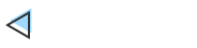两组间差异的非参数检验之Wilcox秩和检验在R中实现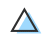在进行两组数据间的差异分析时，我们通常会想到使用t检验。但若数据不满足执行t检验的参数假设(例如数据分布不符合正态性，变量在本质上就严重偏倚或呈现有序关系)，无法使用t检验分析时，可以考虑使用非参数的方法来完成。就两组数据的比较而言，wilcox秩和检验(或称Mann-Whitney U检验)是常见的非参数检验方法之一。本文简介怎样在R中进行wilcox秩和检验，以实现两组间非参数差异分析。本文使用的作图数据的网盘链接(提取码o8lr)：https://pan.baidu.com/s/1b-1INL4HFrsIOvs_0UfByw文件“alpha.txt”为某16S细菌群落测序所获得的部分alpha多样性指数数据，包含3列信息：sample，样本名称；observed_species和shannon分别为两种类型的alpha多样性指数。文件“group.txt”为各样本分组信息，第一列(sample)为各样本名称；第二列(group)为各样本的分组信息。以上使用的示例数据与前文“R语言执行两组间差异分析T检验”中的数据一致。已知group3的shannon指数数据分布并不符合正态性，此时，若我们想比较group2和group3的shannon指数间是否存在显著差异，就不适合使用t检验(暂且不考虑对数据进行合理的转化后是否会满足t检验的参数假设)，可采用非参数的方法(本文中介绍使用wilcox秩和检验)去实现。数据预处理及正态性假设检验首先将上述两个数据表读入R中，并合并在一起，以及数据的正态分布检验。library(reshape2)#读入文件，合并分组信息，数据重排alpha group  alpha  #选择要比较的分组(此处查看 group1 与 group2 在 shannon 指数上是否存在显著差异)shannon_23 shannon_23$group head(shannon_23, 10)#Shapiro-Wilk 检验数据是否符合正态分布(发现不符合正态分布)tapply(shannon_23$value, shannon_23$group, shapiro.test)选取的数据框“shannon_23”内容如下所示。第一列(sample)，两组数据中所含样本名称；第二列(group)，两组分组名称，且分组列已转化为因子类型；第三列(variable)，alpha多样性指数shannon指数；第四列(value)，shannon指数的数值。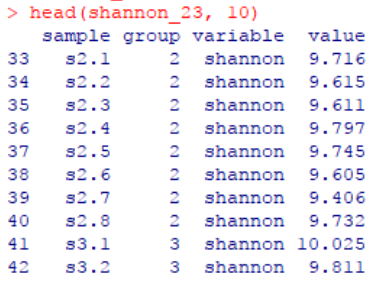通过Shapiro-Wilk检验得知数据分布不满足正态性。这里p值小于0.05表明数据违背了正态性分布的零假设。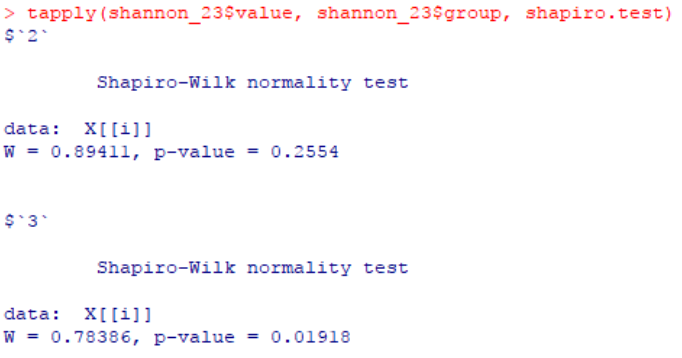Wilcoxon检验不符合正态性前提的数据，无法应用t检验去比较差异。我们考虑使用非参数的方法作为替代，对于两组数据的比较，可使用wilcoxon检验。类似于t检验，根据样本间是否独立，wilcoxon检验分为wilcox秩和检验以及wilcox符号秩和检验。wilcox秩和检验假设样本间是相互独立的，直接使用wilcox秩和检验去处理。它是独立样本t检验的一种非参数替代方法。此处使用的wilcox.test()与t检验t.test()的参数很相似。wilcox_test()中默认两组间相互独立(默认参数paired = FALSE)，执行独立样本的wilcox秩和检验；同时默认的备择假设是双侧的(默认参数alternative = 'two.sided')，即执行双侧检验，可分别使用“alternative = 'less'”或“alternative = 'greater'”执行单侧wilcox检验。##wilcox 秩和检验，我们执行了一个双侧检验wilcox_test wilcox_testwilcox_test$p.value由于p值(约为0.003)小于0.05，即拒绝了原假设(原假设两组间没有差异)，group2和group3的shannon指数间存在显著不同。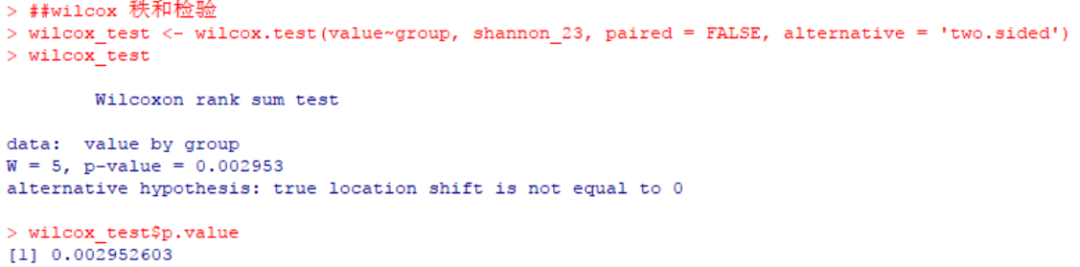wilcox符号秩和检验假设样本间并非相互独立的，可考虑wilcox符号秩和检验，它是非独立样本t检验的一种非参数替代方法。例如，非独立组设计(dependent groups design)、重复测量设计(repeated measures design)等。尽管此时你选用独立样本的wilcox秩和检验方法也是可行的，这种分析方法本身并没问题(仅仅是在统计算法上存在一些不同，相较之下可能wilcox符号秩和检验会更合适一些)。此时在wilcox.test()中设定参数“paired = TRUE”即可执行wilcox符号秩和检验。##wilcox 符号秩和检验，我们执行了一个双侧检验wilcox_test wilcox_testwilcox_test$p.value根据p值(0.039，低于0.05)可知group2和group3的shannon指数间存在显著不同。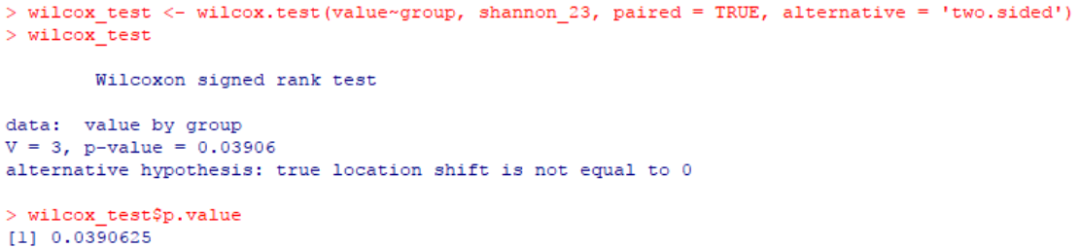可视化展示考虑作图将两组差异进行可视化展示。例如，一个简单的箱线图示例。#boxplot() 箱线图boxplot(value~group, data = shannon_23, col = c('blue', 'orange'), ylab = 'Shannon', xlab = 'Group', main = 'wilcox test: p-value = 0.00295')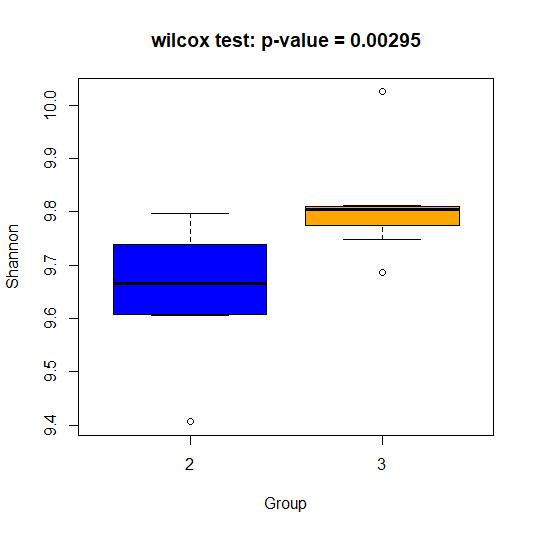Wilcox秩和检验的一个批处理示例相较于参数分析的t检验，wilcox秩和检验不必事先验证数据分布的正态性，因此理论上来讲，只要是两组数据间的差异分析均可使用wilcox秩和检验去完成，因此其适用范围相较于t检验也更广泛。在数据量较大的情况下(可能会存在部分数据满足t检验分析的条件，而另一部分数据则不满足，无法做到全部使用t检验去实现)，可以考虑使用循环逐一挑选分组后，直接执行wilcox秩和检验进行各两两分组间的差异分析。尽管这种方法比较“粗暴”，但也不失为一种备选方案。如下将展示一个批处理示例。网盘附件中提供了另一示例数据集“gene.txt”。表格中每一行为一种基因，每一列为一个样本，交叉区域为各基因在各样本中的相对丰度。接下来，我们期望通过wilcox秩和检验，找到在group1和group2组中，具有丰度差异的基因。##wilcox 检验批处理示例library(doBy) #使用其中的 summaryBy() 以方便按分组计算均值、中位数#读取数据gene group result #使用循环，逐一对各基因进行两组间 wilcox 秩和检验for (n in 1:nrow(gene)) {gene_n gene_id names(gene_n) gene_n$sample gene_n gene_n$group p_value if (!is.na(p_value) & p_value < 0.05) {stat result }}#输出统计结果result names(result) result$p_adjust write.table(result, 'gene.wilcox.txt', sep = '\t', row.names = FALSE, quote = FALSE)我们主要输出这些结果：gene_id，基因id；group1和group2，分别为所需比较的分组1和分组2的名称；mean1、median1、mean2、median2，分别为各基因在分组1、2中的平均丰度以及中位数数值；p_value，显著性p值，此处仅输出了p值低于0.05的结果(即只保留相对丰度在group1、2中具有显著差异的基因)；p_adjust，同时通过Benjamini方法校正p值(嗯嗯，这里的数据p值校正后，没有差异基因……)。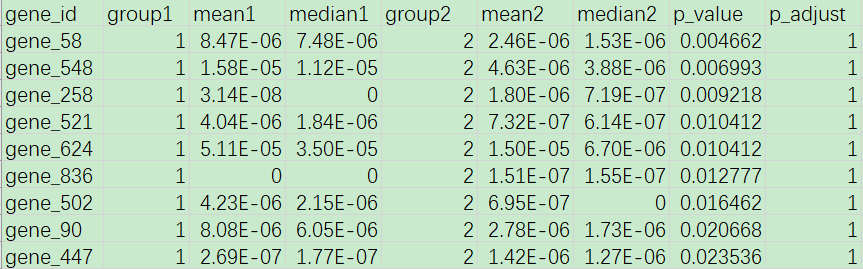特别说明既然参数检验的前提条件有些苛刻，自己的数据不一定都满足参数分析的条件，那么以后需要用到组间差异比较时，直接全部使用非参数的检验就不可以了？虽然对全部数据直接使用非参数的检验方式理论上没啥问题，但还是有点粗暴了一些。两种方法(此处比较了t检验和wilcox秩和检验)毕竟还是有差别的，非参数方法普遍没有参数方法严格。对于符合参数检验条件的数据来讲，使用参数检验还有可能会鉴别出非参数检验鉴别不到的差异，此时需要特别关注。例如，某数据符合t检验的条件，t检验的p值显著，但wilcox检验p值不显著，那么这时t检验的结果会相对可靠一些。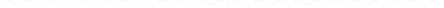友情链接R语言执行两组间差异分析T检验叶绿体基因注释工具PGA叶绿体/线粒体在线注释网站GeSeq线粒体在线注释网站MITOSR语言绘制蝴蝶(柱状)图R语言绘制双向柱状图R语言绘制分组柱状图R语言绘制堆叠面积图R语言绘制堆叠柱状图R语言绘制圆环图R语言绘制饼图(扇形图)R语言绘制花瓣图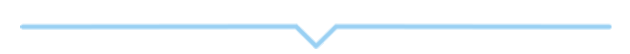展开全文• 卡方检验：主要用于等级资料 。 t检验：适用于计量资料、正态分布、方差...U检验：检验应用条件与t检验基本一致，只是当大样本时用U检验，而小样本时则用t检验，t 检验可以代替U检验。t检验和就是统计量为t,u的假...
卡方检验：主要用于等级资料 。

t检验：适用于计量资料、正态分布、方差具有齐性的两组间小样本比较。包括配对资料间、样本与均数间、两样本均数间比较三种，三者的计算公式不能混淆。也可以这样理解主要是用于小样本（样本容量小于30）的两个平均值差异程度的检验方法。

U检验：检验应用条件与t检验基本一致，只是当大样本时用U检验，而小样本时则用t检验，t 检验可以代替U检验。t检验和就是统计量为t,u的假设检验，两者均是常见的假设检验方法。当样本含量n较大时，样本均数符合正态分布，故可用u检验进行分析。当样本含量n小时，若观察值x符合正态分布，则用t检验（因此时样本均数符合t分布），当x为未知分布时应采用秩和检验。

F检验：则用于方差分析。即对两个或两个以上样本率（构成比）进行差别比较的统计方法。

展开全文卡方检验
• 【—–总体标准差已知时的单个正态总体...% 返回变量h，检验的p值，均值的置信区间muci，检验统计量的观测值zval [h,p,muci,zval] = ztest(x,100,2,0.05) 结果： 由h=1,p=0.0282拒绝原假设 且由置信区间的两个置信
【—–总体标准差已知时的单个正态总体均值的U检验——】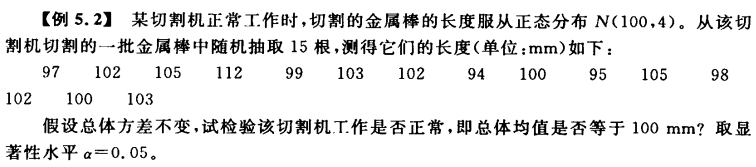（1）分析：建立如下假设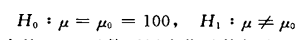（2）matlab进行检验：

% 调用ztest函数作总体均值的双侧检验，
% 返回变量h，检验的p值，均值的置信区间muci，检验统计量的观测值zval
[h,p,muci,zval] = ztest(x,100,2,0.05)   

结果：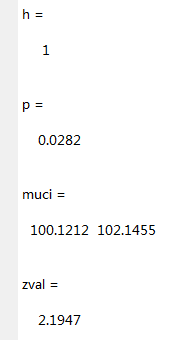由h=1,p=0.0282<0.05拒绝原假设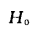且由置信区间的两个置信限都大于100，故作如下假设：：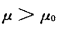=100

% 调用ztest函数作总体均值的单侧检验
[h,p,muci,zval] = ztest(x,100,2,0.05,'left') 

结果：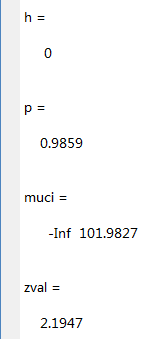由h=0,p=0.9859>0.05接受上述接受即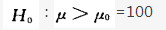所以该切割机不正常，总体均值大于100mm.

【—–总体标准差未知时的单个正态总体均值的t检验—–】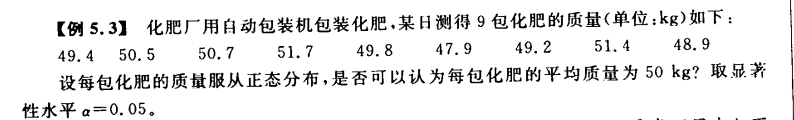（1）分析：建立如下假设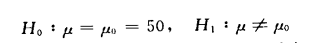（2）matlab进行检验：

x = [49.4  50.5  50.7  51.7  49.8  47.9  49.2  51.4  48.9];    % 定义样本观测值向量
% 调用ttest函数作总体均值的双侧检验，
% 返回变量h，检验的p值，均值的置信区间muci，结构体变量stats
[h,p,muci,stats] = ttest(x,50,0.05)

结果：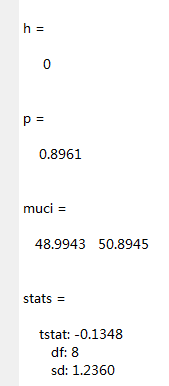由h=0,p=0.8961>0.05知接受上述假设，即每包化肥的平均质量为50kg。

【——总体标准差未知时的两个正态总体均值的比较t检验—–】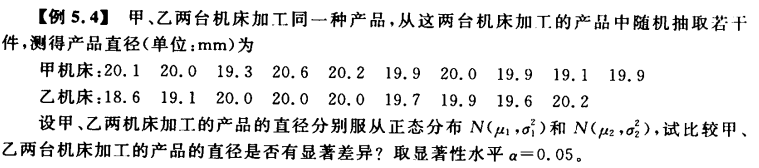（1）分析：建立如下假设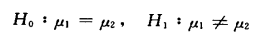（2）matlab进行检验：

% 定义甲机床对应的样本观测值向量
x = [20.1,  20.0,  19.3,  20.6,  20.2,  19.9,  20.0,  19.9,  19.1,  19.9];
% 定义乙机床对应的样本观测值向量
y = [18.6,  19.1,  20.0,  20.0,  20.0,  19.7,  19.9,  19.6,  20.2];
alpha = 0.05;    % 显著性水平为0.05
tail = 'both';    % 尾部类型为双侧
vartype = 'equal';    % 方差类型为等方差
% 调用ttest2函数作两个正态总体均值的比较检验，
% 返回变量h，检验的p值，均值差的置信区间muci，结构体变量stats
[h,p,muci,stats] = ttest2(x,y,alpha,tail,vartype)

结果：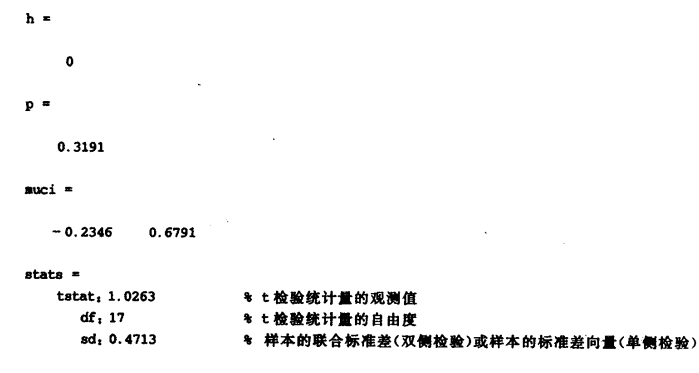由h=0,p=0.3191知接受上述假设，即甲乙两台机床加工的产品的直径没有显著差异。

【——总体均值未知时的单个正态总体方差的卡方检验——】

例5.5：化肥厂用自动包装机包装化肥，某日测得9包化肥的质量（单位：kg）如下：
49.4  50.5  50.7  51.7  49.8  47.9  49.2  51.4  48.9
设每包化肥的质量服从正态分布，是否可以认为每包化肥的质量的方差等于1.5？取显著性水平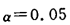（1）分析：建立如下假设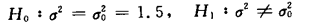（2）matlab进行检验：

% 定义样本观测值向量
x = [49.4  50.5  50.7  51.7  49.8  47.9  49.2  51.4  48.9];
var0 = 1.5;    % 原假设中的常数
alpha = 0.05;    % 显著性水平为0.05
tail = 'both';    % 尾部类型为双侧
% 调用vartest函数作单个正态总体方差的双侧检验，
% 返回变量h，检验的p值，方差的置信区间varci，结构体变量stats
[h,p,varci,stats] = vartest(x,var0,alpha,tail)


结果：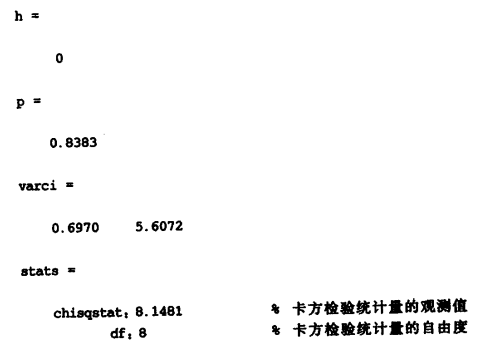由h=0,p=0.8383知接受原假设，即每包化肥的质量的方差等于1.5。

【—总体均值未知时的两个正态总体方差的比较f检验—】

例5.6：甲乙两台机床加工同一种产品，从这两台机床加工的产品中随机抽取若干件，测得产品直径（单位：mm）为
甲机床：20.1,  20.0,  19.3,  20.6,  20.2,  19.9,  20.0,  19.9,  19.1,  19.9
乙机床：18.6,  19.1,  20.0,  20.0,  20.0,  19.7,  19.9,  19.6,  20.2
检验这两台机器机床加工的产品直径的方差是否相等？取显著性水平为0.05.
（1）分析：建立如下假设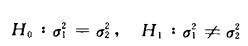（2）matlab进行检验：

% 定义甲机床对应的样本观测值向量
x = [20.1,  20.0,  19.3,  20.6,  20.2,  19.9,  20.0,  19.9,  19.1,  19.9];
% 定义乙机床对应的样本观测值向量
y = [18.6,  19.1,  20.0,  20.0,  20.0,  19.7,  19.9,  19.6,  20.2];
alpha = 0.05;    % 显著性水平为0.05
tail = 'both';    % 尾部类型为双侧
% 调用vartest2函数作两个正态总体方差的比较检验，
% 返回变量h，检验的p值，方差之比的置信区间varci，结构体变量stats
[h,p,varci,stats] = vartest2(x,y,alpha,tail)

结果：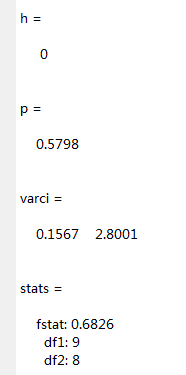由h=0,p=0.5798>0.05知接受原假设，即这两台机器机床加工的产品直径的方差相等。
展开全文• 已知从正态母体N(u,σ2)中抽得容量为n的子样，求得子样的均值x，而且假设母体的方差σ2 为已知值，那么可利用统计量 u = (x -μ) / (σ / √n) ~ N(0,1) 检验母体期望μ是否与某一常数相符进行检...
• 统计检验中的两类错误 在进行假设检验时，分别提出原假设（Null Hypothesis）和备择假设（Alternative Hypothesis），检验结果可能出现的两类错误： 原假设实际上是正确的，而检验结果却拒绝原假设，称为第一类/...
• 在笔者另一篇博客 ( https://blog.csdn.net/Raider_zreo/article/details/101380293 ) 中已经对 Wilcoxon 秩和检验有过介绍，事实上，Wilcoxon 统计量与 Mann-Whitney 统计量是等价的。Wilcoxon 秩和检验主要针对两...
• 在笔者另一篇博客 ( https://blog.csdn.net/Raider_zreo/article/details/101380293 ) 中已经对 Wilcoxon 秩和检验有过介绍，事实上，Wilcoxon 统计量与 Mann-Whitney 统计量是等价的。Wilcoxon 秩和检验主要针对两...python
• 统计推断的另一类重要的问题是假设检验，所谓假设检验就是对总体分布中的某个参数或者分布的形式作出某种假设，利用抽取样本提供的信息，构造适合的统计量，再根据小概率事件进行检验，以作出统计推断。 常用的假设...
• 1．掌握SAS统计量计算、频率分布等描述性统计操作； 2．掌握SAS常用统计图绘制过程步操作； 3. 学习SAS制表过程PROC REPORT； 4．掌握SAS样本均值显著性检验及区间估计的操作。 习题： (一) 在小麦育种时，调查了...
• 科研常见的差异性分析方法汇总根据数据是否符合正态分布，分为：参数检验非参数检验非参数检验是在总体方差未知或知道甚少的情况下，利用样本数据对总体...先由测得的样本数据计算检验统计量，若计算的统计量值落...双因素方差分析
• 假设检验(Hypothesis Testing)是数理统计学中...由实测的样本，计算出统计量的值，并根据预先给定的显著性水平进行检验，作出拒绝或接受假设H0的判断。常用的假设检验方法有u检验法、t检验法、χ2检验法(卡方检...
• 1. 不存在p检验，只有Z检验、T检验、卡方检验、U检验等，这些...同样是t检验，也分为两种：独立样本t检验统计量，配对样本检验。p值的python接口为   p值的python接口为：stats.t.sf(np.abs(tt), n - 1) * 2 ...
• 在国内也被称作u检验。 T检验： 主要用于样本含量较小（例如n < 30），总体标准差σ未知的正态分布。T检验是用t分布理论来推论差异发生的概率，从而比较两个平均数的差异是否显著。 卡方检验： 卡方检验是统计...统计学
• z检验用于检验正态样本均值是否等于某个假设值，不过需要事先知道总体方差，得到的统计量服从正态分布，有的教材上又叫u检验 t检验与z检验相似，t检验不需要知道总体方差，它用样本方差替代总体方差，得到的统计量...
• 之后利用核密度估计的相关知识构造了一个渐近无偏的U统计量来估计该参数, 该检验统计量的值过大时接受随机变量是DRE 的假设. 在一定条件下证明了检验统计量的渐近正态性, 从而得到检验的渐近临界值. 最后确定了核...
• 基本步骤：（1）提出原假设和备择假设 （2）确定适当的检验统计量 （3）规定显著性水平 （4）计算检验统计量的值 （5）做出决策 单样本t 检验：（1）假设样本服从t分布，原假设为总体均值等于u0 （2）备注假设为...
• 假设检验(Hypothesis ...由实测的样本，计算出统计量的值，并根据预先给定的显著性水平进行检验，作出拒绝或接受假设H0的判断。常用的假设检验方法有u-检验法、t检验法、χ2检验法(卡方检验)、F-检验法，秩和检验等。
• 自相关的检验* §6.3 检验自相关的方法 二、杜宾—沃森(Durbin-Watson)检验法 在解析法检验中，用的最多的是杜宾—沃森检验法， 简称D-W检验。 (一) D-W检验的基本思想 对一阶线性...D-W检验是通过构造统计量 (6.3.1...
• 当样本量较小时，渐近统计量和精确检验均具有较低的功效。 我们创建了一组U统计量，并将它们与一些现有统计量进行比较，以测试不同数据设置下OR的同质性。 我们通过蒙托卡洛（Monto Carlo）模拟，根据经验大小和...
• 实际观察次数与理论次数之差的平方再除以理论次数得到的统计量近似服从卡方分布 第四步：查χ2方分布临界值表，确定接受域 一个例子：检验性别和信来世是否独立 另外一种计算卡方值得方法： ...
• 正态总体参数的假设检验统计量 1.总体均值μ\muμ的检验 (1)方差已知-μ\muμ检验 U=n(Xˉ−μ0)σ0U=\frac { \sqrt { n } (\bar { X } -{ \mu }_{ 0 }) }{ { \sigma }_{ 0 } }U=σ0​n​(Xˉ−μ0​)​ 与μ...
• 这种检验过程是通过分析两个独立样本的均值、中位数、离散趋势、偏度等描述性统计量之间的差异来实现的检验类型 Mann-Whitney U检验法 检验两个样本的总体在某些位置上是否相同，其基于对平均秩的分析实现推断。 ...
• 第2章 U统计量 47 2.1 基本概念 48 2.2 U统计量的渐近正态性 54 2.3 多样本U统计量 63 2.4 若干补充知识 68 第3章 秩统计量的极限理论 73 3.1 引言与例子 74 3.2 同分布情况下线性秩统计量的渐近正态性 81 ...
• 应用例子1：一个样本平均数与一个已知的总体平均数的差异 ...提出假设，规定适当检验统计量，确定检验水平： H0：μ=μ0=0.081，H1：μ≠μ0，α=0.05H0：μ=μ0=0.081 ，H1：μ≠μ0，α=0.05H0：μ统计学
• 我最近在研究TCGA的RNAseq数据表达差异性的分析，常用的并且最简单的方法是统计量T检验。下面用一个例子来验证T检验的弊端问题所在。两配对样本的T检验是利用来自两个总体的配对样本，推断总体的两个均值是否显著...数据 R语言 TCGA
• 两总体均值和比例的推断 前面对一个总体的均值和比例...令u1u1u_1和u2u2u_2分别表示总体1和总体2的均值，本节介绍两均值之差：u1−u2u1−u2u_1 - u_2的统计推断 独立简单随机样本：从总体1中抽取一个容量为n1n1n_...统计学
• Wilcoxon signed-rank是一种非参数检验统计量，用于检验对称分布的均值是否为0。给出iid数据Y1,⋯ ,YnY_1,\cdots,Y_nY1​,⋯,Yn​，Zj=sign(Yj)Z_j = sign(Y_j)Zj​=sign(Yj​)，RjR_jRj​为ZjZ_jZj​的秩(rank)....统计学
• u检验是以标准正态分布为理论基础，以U值作为统计量的检验方法，适用于倒数较多的数值资料 T U检验都可用于样本与总体比较，配对设计，两组随机的假设检验 。 方差分析 analysis of variance- ANOVA 它把所有数据...
• 统计量：对数据进行分析检验的变量 (通俗点说就是一种评判方式和标准) 例如：方差，标准差，样本原点矩和中心距，以及最简单的均值。 正态总体下的四大分布 u统计量~标准正态分布 t统计量~t分布 卡方统计量~卡方...机器学习